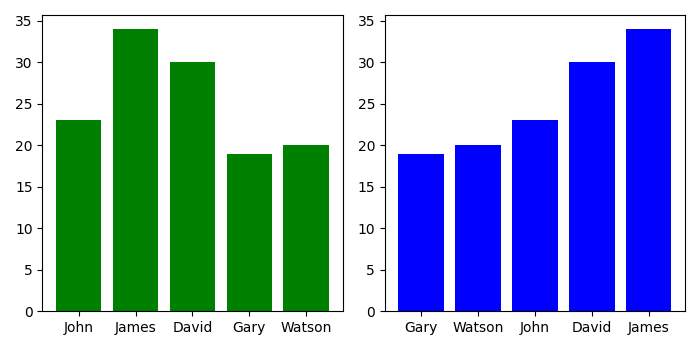# How to determine the order of bars in a matplotlib bar chart?

To determine the order of bars in a bar chart in matplotlib, we can take the following steps −

• Set the figure size and adjust the padding between and around the subplots.
• Make a dataframe, df, of two-dimensional, size-mutable, potentially heterogeneous tabular data.
• Add a subplot to the current figure.
• Make a bar plot with dataframe, df.
• Add a subplot to the current figure.
• Create another dataframe, df_sorted, by column marks.
• Make a bar plot with df_sorted.
• To display the figure, use show() method.

## Example

import pandas as pd
from matplotlib import pyplot as plt

plt.rcParams["figure.figsize"] = [7.00, 3.50]
plt.rcParams["figure.autolayout"] = True

df = pd.DataFrame(
dict(
names=['John', 'James', 'David', 'Gary', 'Watson'],
marks=[23, 34, 30, 19, 20]
)
)

plt.subplot(121)
plt.bar('names', 'marks', data=df, color='green')

plt.subplot(122)
df_sorted = df.sort_values('marks')
plt.bar('names', 'marks', data=df_sorted, color='blue')

plt.show()

## Output

It will produce the following output